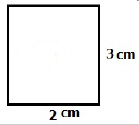3.MD.7.C.-Test1C Show the area of a rectangle with whole-number side lengths
 Name:    3.MD.7.C.-Test1C Show the area of a rectangle with whole-number side lengths

Multiple Choice
Identify the choice that best completes the statement or answers the question.

1.

If a rectangle had an area of 20 sq cm and one side had a length of 4 cm, what would the length of the other side be?
 a. 12 cm c. 16 cm b. 4 cm d. 5 cm

2.

If a rectangle had an area of 4 sq cm and one side had a length of 4 cm, what would the length of the other side be?
 a. 1 cm c. 8 cm b. 2 cm d. 4 cm

3.

If a rectangle had an area of 12 sq cm and one side had a length of 2 cm, what would the length of the other side be?
 a. 6 cm c. 2 cm b. 10 cm d. 14 cm

4.

If a rectangle had an area of 25 sq cm and one side had a length of 5 cm, what would the length of the other side be?
 a. 1 cm c. 5 cm b. 20 cm d. 25 cm

5.

A rectangle has an area of 48 sq cm.  Mark any equation that would describe the length and width of the area.
 a. 2 cm x 24 cm c. 8 cm x c cm b. 16 cm x 2 cm d. 6 cm x 9 cm

6.

A rectangle has an area of 48 sq cm.  Mark any equation that would describe the length and width of the area.
 a. 2 cm x 24 cm c. 8 cm x c cm b. 16 cm x 2 cm d. 6 cm x 9 cm

7.

If a rectangle had an area of 16 sq cm and one side had a length of 4 cm, what would the length of the other side be?
 a. 12 cm c. 6 cm b. 4 cm d. 20 cm

8.

What is the area of this rectangle?a. 10 cm c. 6 cm b. 3 cm d. 5 cm

9.

If a rectangle had an area of 24 sq cm and one side had a length of 4 cm, what would the length of the other side be?
 a. 3 cm c. 6 cm b. 28 cm d. 20 cm

Multiple Response
Identify one or more choices that best complete the statement or answer the question.

10.

If a rectangle had an area of 36 sq cm, what are the possible width/length measurements?
 a. 1 cm x 36 cm c. 3 cm x 12 cm b. 9 cm x 4 cm d. 18 cm x 2 cm